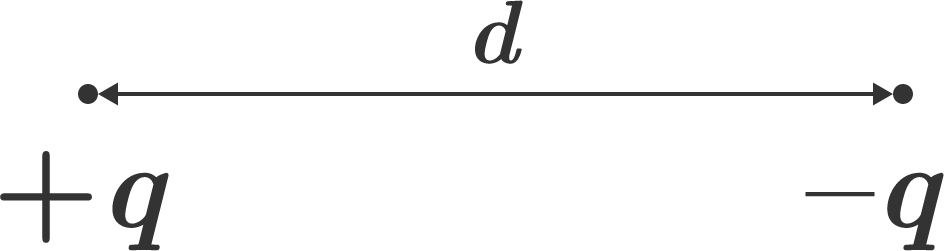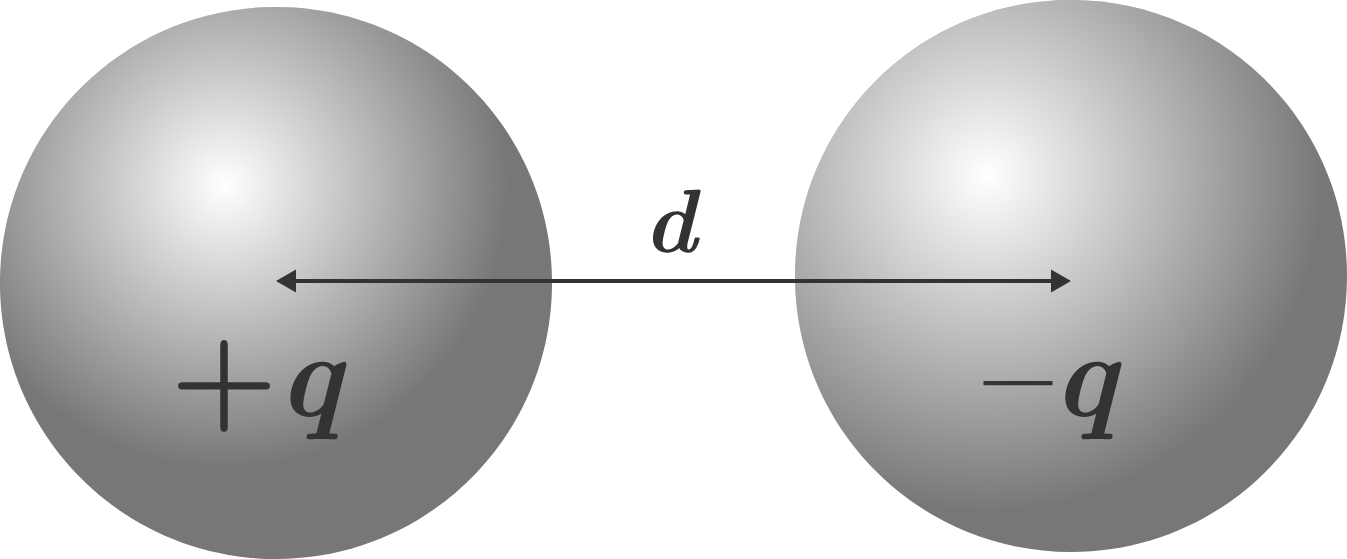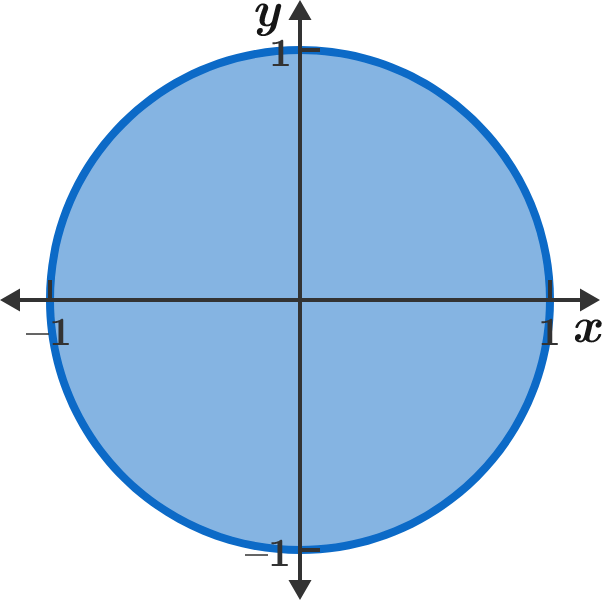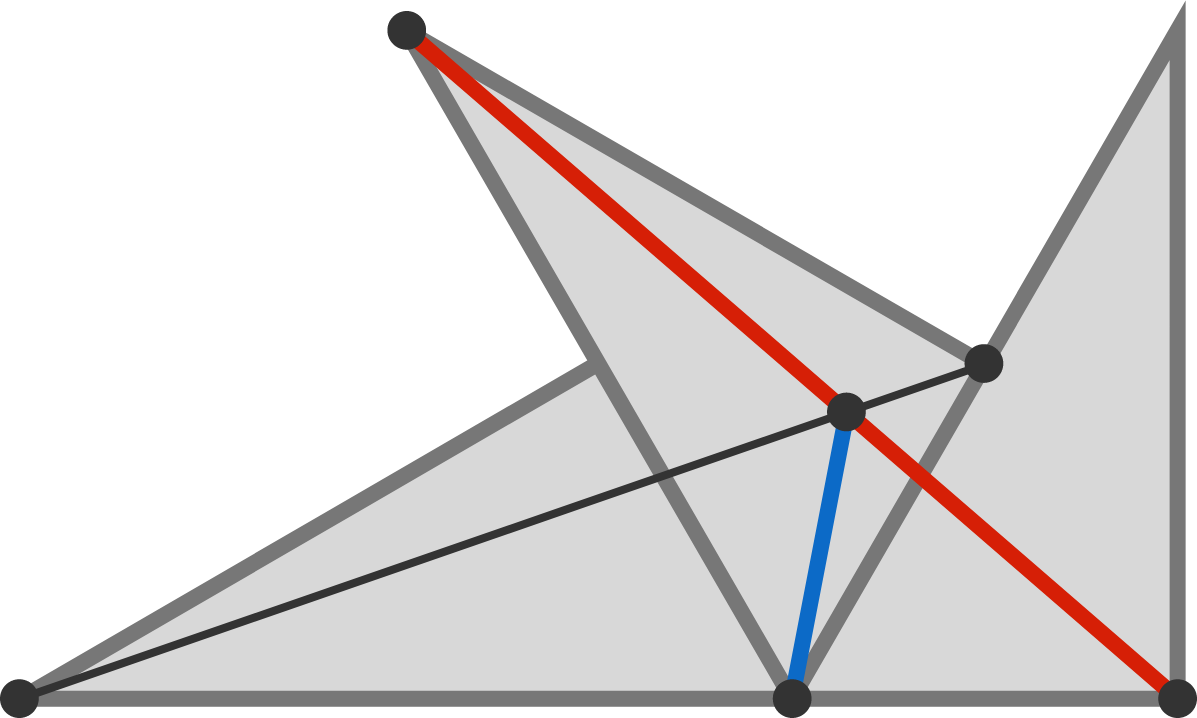Problems of the Week

Contribute a problem

2018-01-08 IntermediateIf two point charges $+q$ and $-q$ are separated by distance $d,$ then according to Coulomb's law, the attractive force between them is

$F_e=k_e\frac{q^2}{d^2},$

where $k_e$ is Coulomb's constant. But what if the charges were distributed on spheres instead of at points? Two charged conducting spheres with charges $+q$ and $-q$ are separated by center-to-center distance $d.$The attractive force between them is $\text{\_\_\_\_\_\_\_\_\_\_}.$

 $n$ $\hspace{10mm} 2^n$ Concatenation of the powered numbers Divisibility checked 0 $\hspace{5mm} 2^0=1$ $\hspace{25mm} 1$ $\hspace{8mm} 1\, \big|\, 1$ 1 $\hspace{5mm} 2^1=2$ $\hspace{25mm} 12$ $\hspace{8mm} 2\, \big|\, 12$ 2 $\hspace{5mm} 2^2=4$ $\hspace{25mm} 124$ $\hspace{8mm} 4\, \big|\, 124$ 3 $\hspace{5mm} 2^3=8$ $\hspace{25mm} 1248$ $\hspace{8mm} 8\, \big|\, 1248$ 4 $\hspace{5mm} 2^4=16$ $\hspace{25mm} 124816$ $\hspace{8mm} 16\, \big|\, 124816$

As we get greater and greater numbers in column 3 of the table by concatenation (i.e. 12481632, 1248163264, ...) for $n>4,$ will the divisibility in the last column still hold?It's common knowledge that the graph of $x^2 + y^2 \leq 1$ is a unit disk, and the area of the region is $\pi.$

However, it's not common for someone to know the shape of the graph $\big\lfloor x^2 \big\rfloor + \big\lfloor y^2 \big\rfloor = 1,$ where $\lfloor \cdot \rfloor$ is the floor function. To three decimal places, what is the area of the region on the coordinate plane that satisfies the equation $\big\lfloor x^2 \big\rfloor + \big\lfloor y^2 \big\rfloor = 1?$


Note: Try to draw a hand sketch of this curve and provide analysis instead of using any software!

Does there exist a function $f: \mathbb{R} \rightarrow \mathbb{R}$ such that for every two distinct real numbers $a$ and $b,$ $f(a)$ and $f(b)$ differ by at least $1?$Three identical 30-60-90 right triangles are arranged as shown in the figure.

What is the ratio of the $\color{#D61F06}\text{red segment's}$ length to the $\color{#3D99F6}\text{blue segment's}$ length?

×